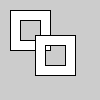# Py5Graphics.begin_contour()#

Use the begin_contour() and Py5Graphics.end_contour() methods to create negative shapes within shapes such as the center of the letter ‘O’.

## Examples#def setup():
py5.size(100, 100, py5.P2D)

g = py5.create_graphics(60, 60, py5.P2D)
with g.begin_draw():
with g.begin_closed_shape():
g.vertex(10, 10)
g.vertex(50, 10)
g.vertex(50, 50)
g.vertex(10, 50)
with g.begin_contour():
g.vertex(20, 20)
g.vertex(20, 40)
g.vertex(40, 40)
g.vertex(40, 20)

py5.image(g, 0, 0)
py5.image(g, 25, 25)


## Description#

Use the begin_contour() and Py5Graphics.end_contour() methods to create negative shapes within shapes such as the center of the letter ‘O’. The begin_contour() method begins recording vertices for the shape and Py5Graphics.end_contour() stops recording. The vertices that define a negative shape must “wind” in the opposite direction from the exterior shape. First draw vertices for the exterior shape in clockwise order, then for internal shapes, draw vertices counterclockwise.

These methods can only be used within a Py5Graphics.begin_shape() & Py5Graphics.end_shape() pair and transformations such as Py5Graphics.translate(), Py5Graphics.rotate(), and Py5Graphics.scale() do not work within a begin_contour() & Py5Graphics.end_contour() pair. It is also not possible to use other shapes, such as Py5Graphics.ellipse() or Py5Graphics.rect() within.

This method can be used as a context manager to ensure that Py5Graphics.end_contour() always gets called, as shown in the example.

This method is the same as begin_contour() but linked to a Py5Graphics object.

Underlying Processing method: PGraphics.beginContour

## Signatures#

begin_contour() -> None


Updated on March 06, 2023 02:49:26am UTC